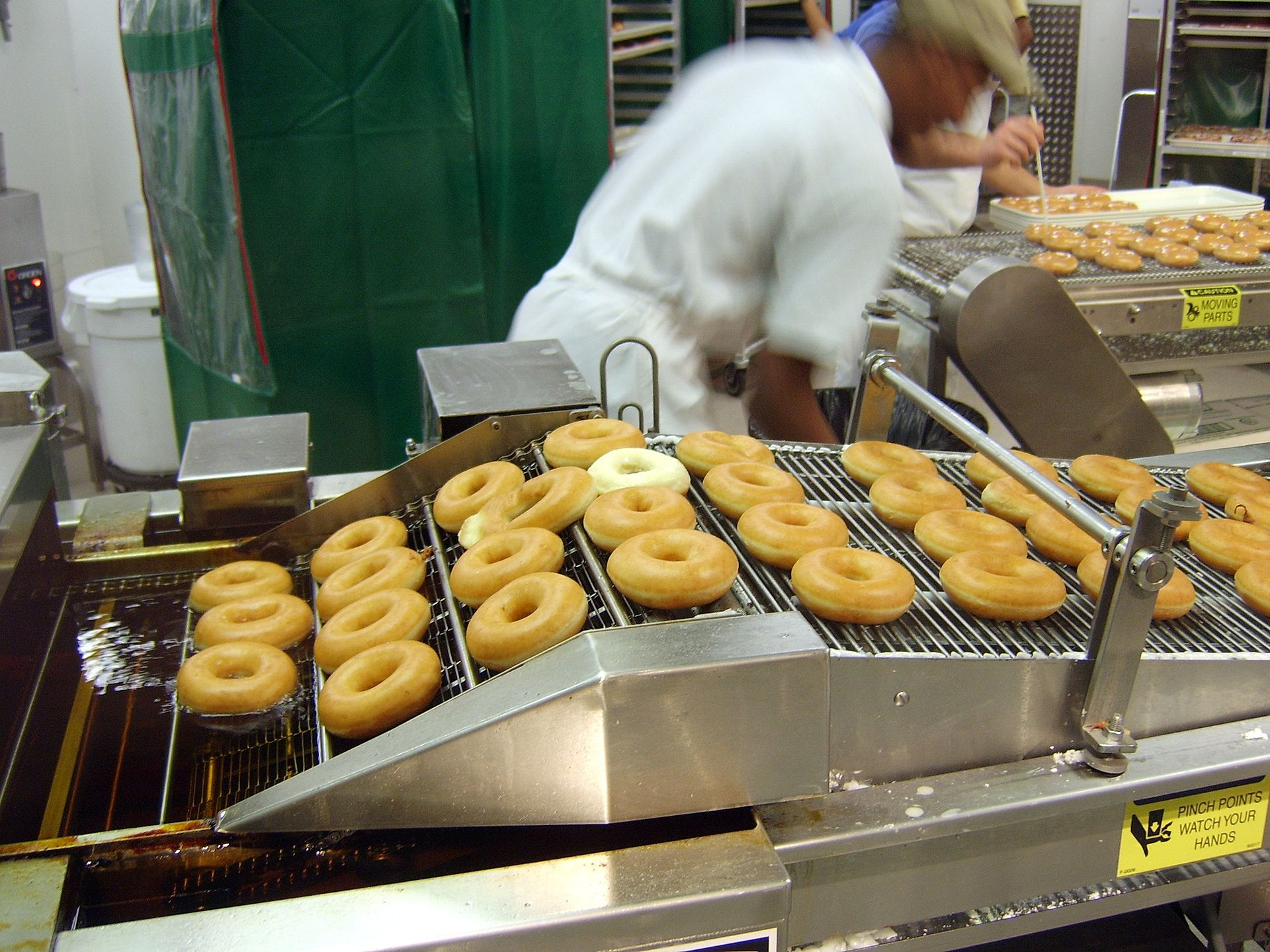Doughnut Production Line by Kozuch, licensed under the Creative Commons Attribution-Share Alike 2.0 Generic license.

You are given a work list that must be processed in the given order in such a way that no two identical items are processed within a given cooldown period. Compute the amount of time that takes processing the work list.

Processing each item takes one time unit and the cooldown period is given in time units. When a given item is within the cooldown of another identical item, do nothing until the cooldown period elapses.

Consider work list AABACBC and cooldown period of 2 time units. The processing of the work list is the following.

Input. The input consists of one or more cases. Each case consists of two lines. The first line of a case consists of a single integer, the time units in the cooldown period. The second line of a case consists of a string, the work list. The input is terminated by EOF. The following is a sample input file.

Output. For each input case, print on a single line the time units it takes to process the work list. The following is the output file that corresponds to the sample input file.

# Solution

Given a work list of length n, the proposed solution takes O(n) time and O(1) space.

Consider the processing of work list AABAB with cooldown period of 2 time units.

The cooldown period indicates the minimum separation between any two identical items. When two identical items are not far enough, we introduce the minimum amount of idle time slots possible to satisfy the cooldown period. For example, between the first and second A we introduce two idle time slots because there is nothing in between them. Another example, for the second and third A, we introduce one idle time slot because there is already the time slot of B between the two A. Another example, by the time we start processing the second B, the distance to the first B is already more than the cooldown period, so we do not introduce idle time.

When two identical work items are within the cooldown time, the amount of idle time slots we introduce is the difference between the cooldown period and the current distance between the items. We introduce the idle time before the second work item. For example, we introduce one idle slot before the third A because the distance between the time when we finish processing the first B and the time we finish processing the first A is 1 as illustrated in the following diagram.

To compute the distance between one item and the previous identical item, we store in a hash the current time for the current element as illustrated in the following diagram.

Given hash last, current work item w, and current time time, the computation of the distance is given by expression time - last[w].